HistoryMathematiciansAll formulasNum. approx.SoftwaresMisc. math.DigitsPoetryPapers/videosDelirium !Pi-DayImagesMusicLinksBibliography Boris Gourévitch The world of Pi - V2.57 modif. 13/04/2013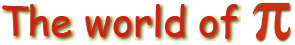HomeVersion historyGuestbookWho I amSome pictures (fr)AcknowledgementsLast modificationsContact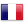Benoit Cloitre

e and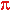in a mirror

Benoit Cloitre is a brilliant maths amateur when he wants.

1 Two mirrored sequences

Consider the sequences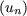n and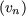n to be defined as

 u1= 0, u2 = 1, un+2 = un+1 +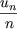v1= 0, v2 = 1, vn+2 =+ vn
ThenIn general, for

 u1= x, u2 = y, un+2 = un+1 +Then

lim n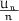= x +By considering those two sequences, e and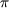are mirrored! However, the speed of convergence are very different... Un converges very quickly (faster and faster) while V n converges in a logarthmic way...

2 Prove

Benoit Cloitre first offered a direct prove, but one can prefer Gery Huvent's prove, from Lille, which allow us to find a general method to prove those sequences.

2.1 Forn

Calculating the first few terms 0,1,1,,,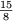,suggest thatThis property can be prove by induction on p, since it's true for p = 1. Suppose that v2p = v2p+1 then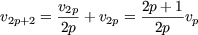and

 v2p+3 =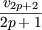+ v2p+1 =*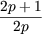vp + v2p =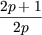vp = v2p+2
hence the result. We also notice that the sequence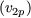p satisfywhich by induction give usTaking into account the classical equivalence (obtain with Wallis)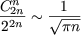we have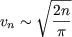Note We can find a limited development with Stirling's formula, to get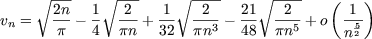2.2 Forn

Consider the function that generates the sequencenthe given recurrent sequence can be written asor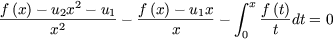by differentiating, we get= 0

hence f is the solutiong to the differential equation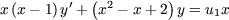which has the general solution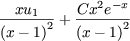taking into acount than u1 = 0 and u2 = 1 =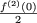we findNow we need to determine the development of the power serie of f so to find each term of the sequence. For this note that(= v2x + v3x2 + ....is defined in 0 )
Then, we remember that if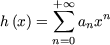then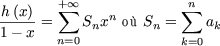using Cauchy's product of two series. Hence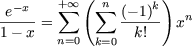andand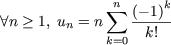more particularlyNote If we did the same for the sequence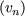n, the generating function is a solution to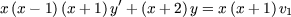which satisfy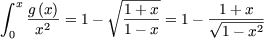with